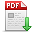# 马吉明,张嵩,苏日建,等.一种元启发式算法--海岛算法[J].郑州大学学报(工学版),2019,40(04):10. 　A metaheuristic algorithm--island algorithm[J].Journal of Zhengzhou University (Engineering Science),2019,40(04):10. 点击复制 一种元启发式算法--海岛算法() 分享到： var jiathis_config = { data_track_clickback: true };

40

2019年04期

10

2019-07-10

## 文章信息/Info

Title:
A metaheuristic algorithm--island algorithm

A

Abstract:
Under the assumption that the total amount of plants is constant, the locations of plants on the island become more and more concentrated at the highest point with the rising of sea level. Inspired by this phenomenon, a metaheuristic algorithm, Island algorithm (IA), is proposed. The Island algorithm consists of three phases in each iteration: elimination phase, sea level rising phase and balance phase. By analyzing the IA algorithm, the reason of the algorithm advantage, the characteristics of the algorithm and the characteristics of the suitable and unsuitable functions are found out. The complexity and robustness of IA algorithm are analyzed.. The algorithm is applied to eight typical test functions in many dimensions and compared with the PSO algorithm and BA algorithm. The results show that IA algorithm is slightly worse than PSO algorithm and BA algorithm in calculating functions with certain characteristics; In the results of the other test functions, the accuracy and robustness of the algorithm are significantly better than PSO algorithm and BA algorithm in many dimensions, which verifies the analysis of IA algorithm.